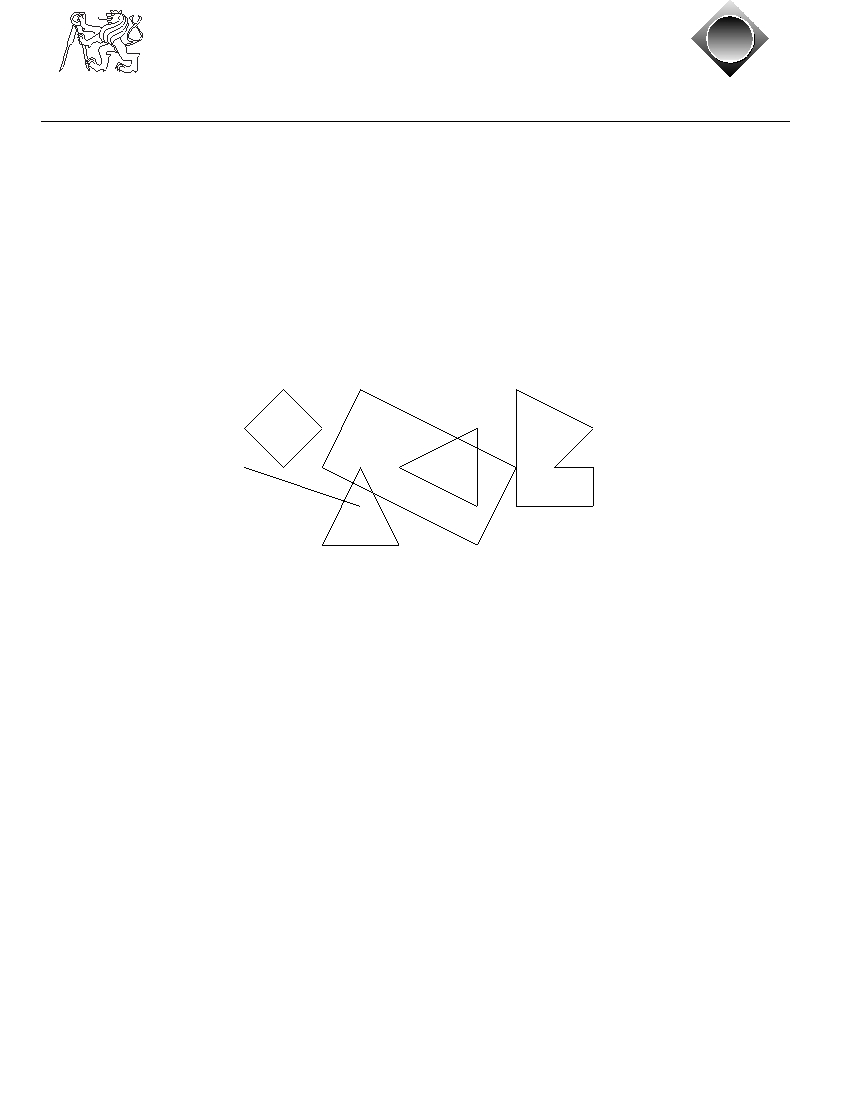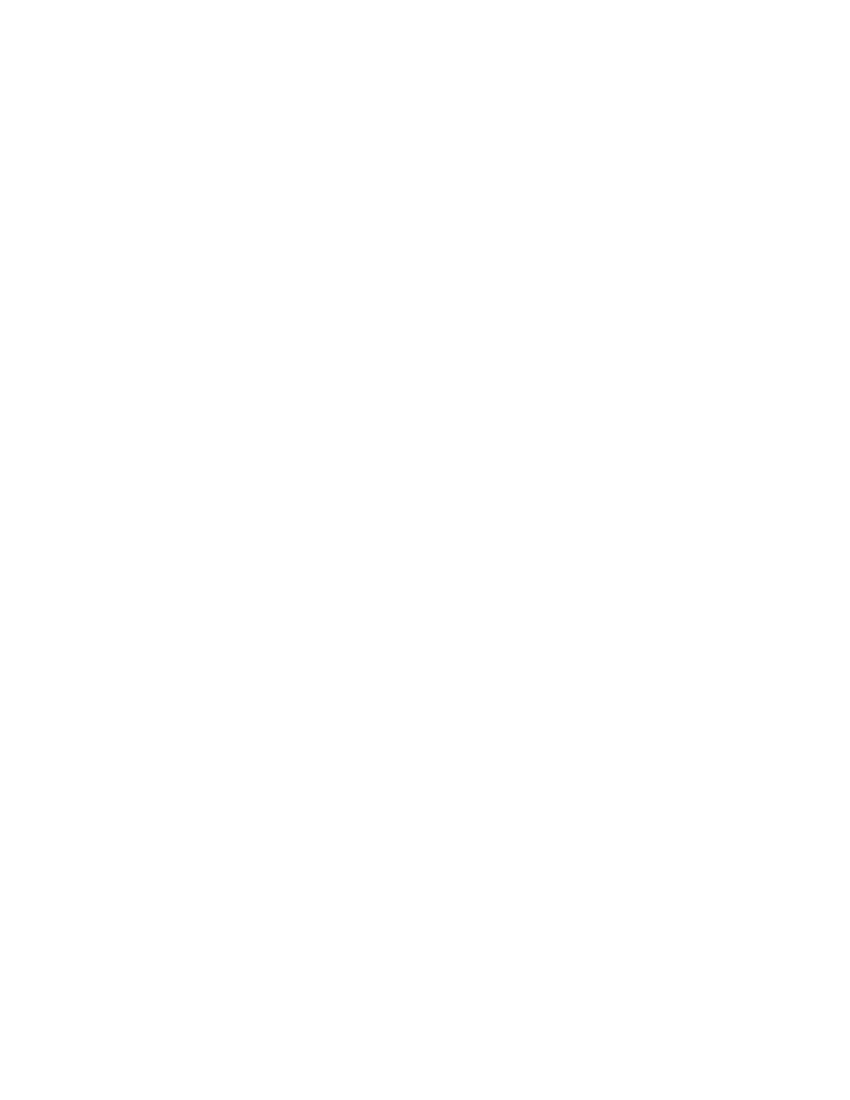Czech ACM Student Chapter
Czech Technical University in Prague
Charles University in Prague
Technical University of Ostrava
Slovak University of Technology
Masaryk University
acm
cz
acm
cz
CTU Open Contest 2007
Geometric Shapes
geometry.c, geometry.C, geometry.p, geometry.java
While creating a customer logo, ACM uses graphical utilities to draw a picture that can later
be cut into special .uorescent materials. To ensure proper processing, the shapes in the picture
cannot intersect. However, some logos contain such intersecting shapes. It is necessary to detect
them and decide how to change the picture.
Given a set of geometric shapes, you are to determine all of their intersections. Only outlines
are considered, if a shape is completely inside another one, it is not counted as an intersection.
F
X
W
A
B
S
Input Specification
Input contains several pictures. Each picture describes at most 26 shapes, each specified on
a separate line. The line begins with an uppercase letter that uniquely identifies the shape
inside the corresponding picture. Then there is a kind of the shape and two or more points,
everything separated by at least one space. Possible shape kinds are:
• square: Followed by two distinct points giving the opposite corners of the square.
• rectangle: Three points are given, there will always be a right angle between the lines
connecting the first point with the second and the second with the third.
• line: Specifies a line segment, two distinct end points are given.
• triangle: Three points are given, they are guaranteed not to be co-linear.
• polygon: Followed by an integer number N (3 . N . 20) and N points specifying vertices
of the polygon in either clockwise or anti-clockwise order. The polygon will never intersect
itself and its sides will have non-zero length.
All points are always given as two integer coordinates X and Y separated with a comma and
enclosed in parentheses. You may assume that |X|, |Y |.10000.
The picture description is terminated by a line containing a single dash ("-"). After the last
picture, there is a line with one dot (".").
pg_0002Output Specification
For each picture, output one line for each of the shapes, sorted alphabetically by its identifier
(X). The line must be one of the following:
• "X has no intersections", if X does not intersect with any other shapes.
• "X intersects with A", if X intersects with exactly 1 other shape.
• "X intersects with A and B", if X intersects with exactly 2 other shapes.
• "X intersects with A, B, ..., and Z", if X intersects with more than 2 other shapes.
Please note that there is an additional comma for more than two intersections. A, B,etc. are
all intersecting shapes, sorted alphabetically.
Print one empty line after each picture, including the last one.
Sample Input
A square (1,2) (3,2)
F line (1,3) (4,4)
W triangle (3,5) (5,5) (4,3)
X triangle (7,2) (7,4) (5,3)
S polygon 6 (9,3) (10,3) (10,4) (8,4) (8,1) (10,2)
B rectangle (3,3) (7,5) (8,3)
-
B square (1,1) (2,2)
A square (3,3) (4,4)
-
.
Output for Sample Input
A has no intersections
B intersects with S, W, and X
F intersects with W
S intersects with B
W intersects with B and F
X intersects with B
A has no intersections
B has no intersections